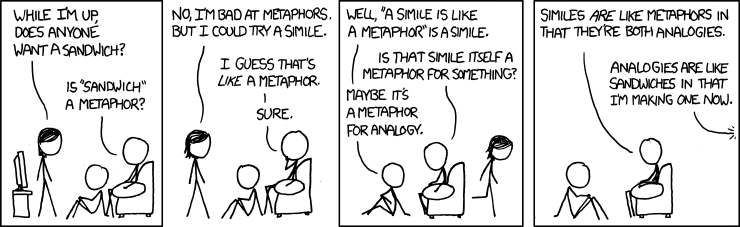# This title is like an analogy; it should explain this post but it really doesn’t.

Title: How should textbook analogies be used in teaching physics?
Authors: Nilüfer Didiş Körhasan and Mustafa Hidir
First author’s institution: Bülent Ecevit University, Turkey
Journal: Physical Review Physics Education Research, 15 010109 (2019)

Analogies are used all of the time in physics education. From using the planets orbiting the sun to explain how electrons orbit the nucleus to using spots on a balloon being inflated to explain the Big Bang and the expansion of the universe, it is almost inconceivable that a physics course could be taught without using some type of analogies. Yet, when using analogies to explain concepts, it is important to make sure that students don’t over-apply the analogy and develop alternative conceptions of the phenomenon. For example, if a student were to over-apply the balloon analogy, they may believe that the universe is expanding into something else just like the balloon expands into the air around it rather than the expansion of the universe being caused by space itself expanding. Today’s paper seeks to understand how analogies are used in science textbooks and what can be done to make them more useful.

### Background

The Teaching-with-analogies (TWA) approach by Glynn is a well-known approach for understanding how analogies are used in science courses. In this approach for describing a “good” analogy, the analogy should begin by introducing the new phenomenon, which in this approach is referred to as the target. Next, the analogy should remind students of the important features of the phenomenon they already understand, referred to as the analog. The analogy should then describe the similarities of the new and old phenomenon and draw conclusions. The analogy should end by describing the limitations of the analogy to ensure that students do not overextend it.

This approach can then be summarized in three steps: i) the pretopic orientation in which the analogy introduces the new phenomenon and the relevant details about the already understood phenomenon ii) the level of enrichment in which the analogy explains the similarities between the new and old phenomenon and iii) the limitations of the analogy in which the analogy explains where it breaks down. An example of this approach applied to the photoelectric effect can be seen in figure 1 in which the photoelectric effect is compared to asking a parent for permission to do something.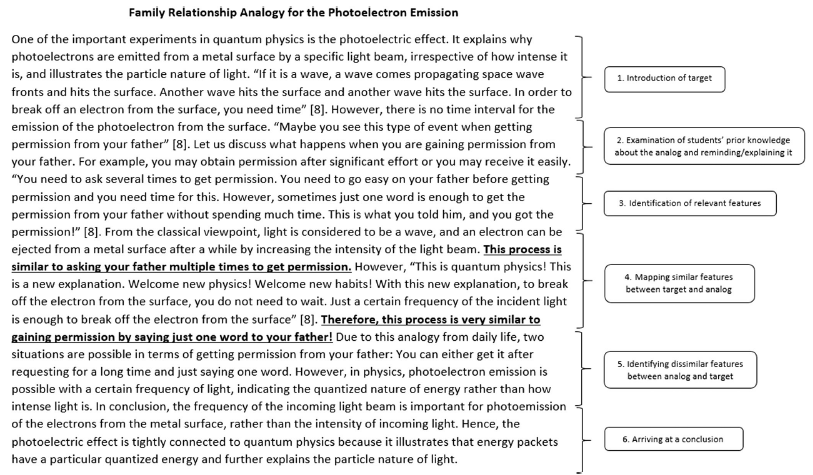Figure 1: A sample analogy for the photoelectric effect with relevant parts relating to the three steps listed above annotated (fig 1 in paper).

### Methods

The researchers then used these three steps as a guide to rating the effectiveness of analogies. They began by selecting six science textbooks approved for use in 3rd-8th grade courses by the Turkish Ministry of Education. They used content analysis and identified examples of analogies in the textbooks. As concepts can be explained with examples, models, analogies, or other methods, the researchers needed to identify when an analogy was the method used to explain the concept and only focus on those cases. Each analogy was then evaluated based on whether it contained the three steps of the approach. An analogy that contained all of the steps was categorized as “good,” an analogy with only one or two of the steps was categorized as “moderate,” and an analogy with none of the steps was categorized as “poor.”

### Results

Across the six textbooks, the researchers identified 141 analogies. Of these, 54 or 38.3% were rated as “poor,” 86 or 61% were rated as “moderate,” and only 1, corresponding to 0.7% of the analogies, was rated as “good.” Overall, analogies were most often used to explain physics concepts (43% of the total analogies) as opposed to chemistry, biology, or engineering concepts. Of these physics analogies, most (54%) were rated as “poor.” In contrast, 75% of the chemistry analogies, 72.3% of biology analogies, and 100% of engineering analogies were rated as “moderate.”

#### Improvements

Next, the researchers focused on two “moderate” examples of physics analogies. The first example from one of the textbooks studied used an analogy between the attraction of two magnets and the gravitational force (figure 2).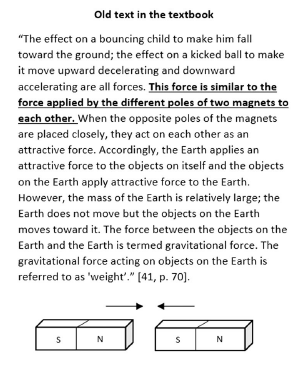Figure 2: A physics analogy found in one of the studied textbooks. This analogy is missing a statement of limitations and a pretopic orientation (fig 3 in paper).

In this textbook analogy, there is no pretopic orientation explaining the attraction between magnets or a statement of limitations about how the magnetic attraction is different from the gravitational attraction. The revised analogy is shown in figure 3, in which the magnetic attraction is further explained and a key limitation is addressed: the magnetic attraction can be attractive or repulsive while the gravitational force can only be attractive.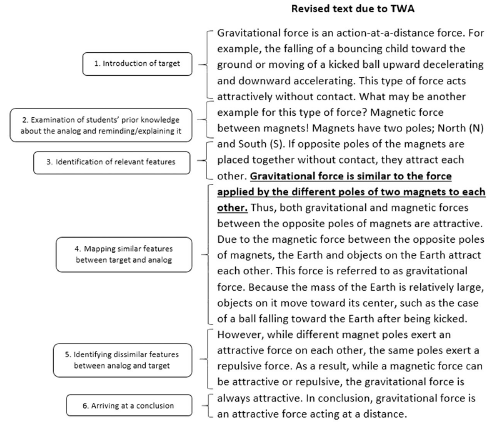Figure 3: Revised analogy for gravitational attraction shown in figure 2. Notice the steps are outlined in the margin (figure 3 in paper).

The second textbook analogy revised was the often-used comparison of water flowing through a pipe to energy flowing in an electrical circuit (figure 4).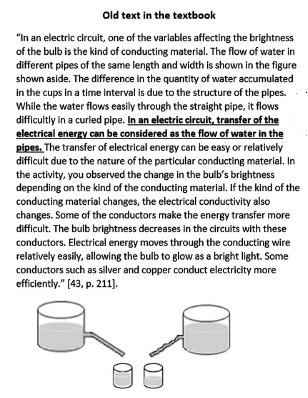Figure 4: A physics analogy found in one of the textbooks studied. This example lacks a statement of limitations (fig 4 in paper).

In this original analogy, there is no explanation of how the flow of energy and of water is different. In the revised analogy (figure 5), this limitation is highlighted to emphasize that nothing is actually flowing in the circuit but rather that energy is transferred. By making this limitation explicit, the revised analogy can dispel the alternative conception that the electrons flow at the speed of light and hence, a light bulb turns on immediately when the switch is flipped. Instead, the analogy makes it clear that it is the field (and hence the energy) that actually moves at the speed of light.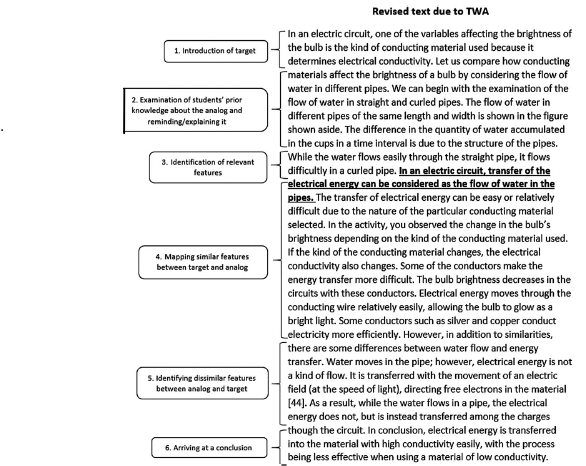Figure 5: The revised analogy for electrical circuits. The steps are shown in the margins. (Fig 4 in the paper).

### Takeaways

The elementary school textbooks used in this study did not meet the criteria of good analogies, often because they did not address the limitations of the analogies. To create more useful analogies, instructors should review analogies in their lessons and textbooks and ensure that the analogy contains a pretopic orientation, levels of enrichment, and a discussion of the limits of the analogy. Upon finding an analogy lacking in any of these areas, the instructor should supplement the analogy with the missing steps before using it in their classrooms.